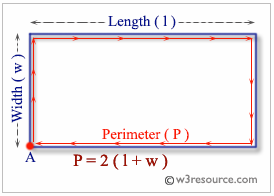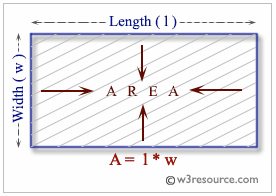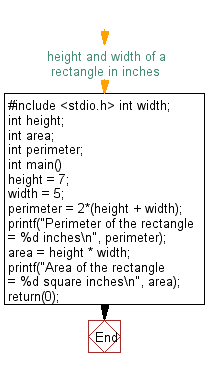﻿ C Program: Compute the perimeter and area of a rectangle - w3resource

# C Exercises: Compute the perimeter and area of a rectangle

## C Basic Declarations and Expressions: Exercise-5 with Solution

Write a C program to compute the perimeter and area of a rectangle with a height of 7 inches and width of 5 inches.

C programming: Perimeter of a rectangle

A perimeter is a path that surrounds a two-dimensional shape. The word comes from the Greek peri (around) and meter (measure). The perimeter can be used to calculate the length of fence required to surround a yard or garden. For rectangles or kites which have only two different side lengths, say x and y, the perimeter is equal to 2x + 2y

Pictorial Presentation:C programming: Area of a rectangle

The area of a two-dimensional figure describes the amount of surface the shape covers. You measure area in square units of a fixed size, square units of measure are square inches, square centimeters, or square miles etc. The formula for the area of a rectangle uses multiplication: length • width = area.  A rectangle with four sides of equal length is a square.

Pictorial Presentation:C Code:

``````#include <stdio.h>

/*
Variables to store the width and height of a rectangle in inches
*/
int width;
int height;

int area;           /* Variable to store the area of the rectangle */
int perimeter;      /* Variable to store the perimeter of the rectangle */

int main() {
/* Assigning values to height and width */
height = 7;
width = 5;

/* Calculating the perimeter of the rectangle */
perimeter = 2*(height + width);
printf("Perimeter of the rectangle = %d inches\n", perimeter);

/* Calculating the area of the rectangle */
area = height * width;
printf("Area of the rectangle = %d square inches\n", area);

return(0);
}
``````

Explanation:

In the exercise above -

• The program includes the standard input/output library <stdio.h>.
• It declares several integer variables:
• width: Represents the rectangle width in inches.
• height: Represents the height of the rectangle in inches.
• area: Will store the calculated rectangle area.
• perimeter: Will store the calculated rectangle perimeter.
• In the "main()" function:
• It assigns the values 7 to the 'height' variable and 5 to the 'width' variable, representing the rectangle's dimensions.
• The program then calculates the rectangle's perimeter and area:
• Perimeter: It uses the formula 2 * (height + width) to calculate the perimeter of the rectangle and stores the result in the 'perimeter' variable.
• Area: It calculates the area using the formula height * width and stores the result in the 'area' variable.
• Finally, the program uses the "printf()" function to display the calculated values:
• It prints the calculated perimeter with a message: "Perimeter of the rectangle = [perimeter] inches."
• It prints the calculated area with a message: "Area of the rectangle = [area] square inches."
• The program returns 0 to indicate successful execution.

Sample Output:

```Perimeter of the rectangle = 24 inches
Area of the rectangle = 35 square inches
```

Flowchart:C Programming Code Editor:

What is the difficulty level of this exercise?

Test your Programming skills with w3resource's quiz.

﻿